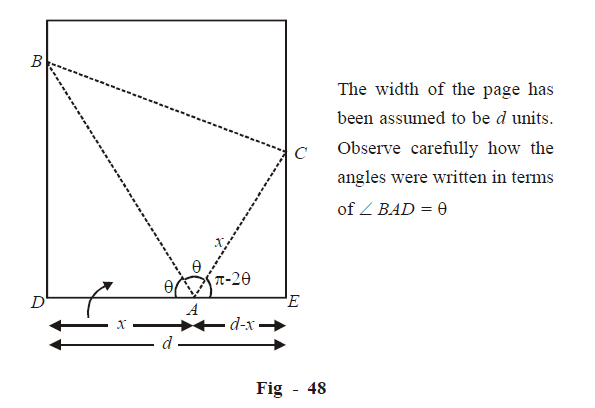# Applications of Derivatives Set 6

Go back to  'SOLVED EXAMPLES'

Example - 12

Let \begin{align}f\left( x \right) = {\sin ^3}x + \lambda {\sin ^2}x\,\,{\rm{for}}\,\,\frac{{ - \pi }}{2} < x < \frac{\pi }{2}.\end{align} Find the interval in which $$\lambda$$ should lie in order that $$f\left( x \right)$$ has exactly one minimum and one maximum.

Solution:  We have to equivalently find those values of $$\lambda$$ for which $$f'\left( x \right)$$ has two roots; $$f''\left( x \right)$$ should be positive for one root and negative for the other.

\begin{align}&f'\left( x \right) = 3{\sin ^2}x\cos x + 2\lambda \sin x\cos x\\\\&\qquad \;= \sin 2x\left( {\lambda + \frac{3}{2}\sin x} \right)\end{align}

\begin{align}For\; x \in \left( {\frac{{ - \pi }}{2},\frac{\pi }{2}} \right),f'\left( x \right)\,{\text {is}}\,\,0 \,\,when:\end{align}

\begin{align}x = 0,\,\,\,{\sin ^{ - 1}}\left( {\frac{{ - 2\lambda }}{3}} \right)\end{align}

Note that if \begin{align}\lambda = 0,\,\,{\sin ^{ - 1}}\left( {\frac{{ - 2\lambda }}{3}} \right) = 0\end{align} so that the two roots of $$f'\left( x \right)$$will no longer remain distinct and$$f\left( x \right)$$will not have two extremum points as required. Hence, $$\lambda$$should not be 0.

For                              \begin{align}{\sin ^{ - 1}}\left( {\frac{{ - 2\pi }}{3}} \right)\end{align} to be defined:

\begin{align} - 1 < \frac{{ - 2\lambda }}{3} < 1\\\\\frac{{ - 3}}{2} < \lambda < \frac{3}{2}\end{align}

Therefore,   \begin{align}\lambda \in \left( {\frac{{ - 3}}{2},\,\frac{3}{2}} \right)\backslash \left\{ 0 \right\}\end{align}

To verify that  $$f\left( x \right)$$  will satisfy the required condition for these values of  $$\lambda$$, let us evaluate  $$f''\left( x \right)$$

\begin{align}&f''\left( x \right) = \frac{3}{2}\cos x\sin 2x + \left( {3\sin x + 2\lambda } \right)\cos 2x\\\\ &\Rightarrow \quad f''\left( 0 \right) = 2\lambda \,\,and\,\,{\left. {f''\left( {{{\sin }^{ - 1}}\left( {\frac{{ - 2\lambda }}{3}} \right)} \right) = \frac{3}{2}\cos x\sin 2x} \right|_{{{\sin }^{ - 1}}\left( {\frac{{ - 2\lambda }}{3}} \right)}}\\\\&\,\,\,\,\,\,\,\,\,\,\,\,\,\,\,\,\,\,\,\,\,\,\,\,\,\,\,\,\,\,\,\,\,\,\,\,\,\,\,\,\,\,\,\,\,\,\,\,\,\,\,\,\,\,\,\,\,\,\,\,\,\,\left\{ \begin{gathered}{\text {The }}\,{\text{second term in }}f''\\\\{\text{becomes 0 at this point}}\end{gathered} \right\}\\\\&Now,{\text{ }}if\,\,\lambda \in \left( {\frac{{ - 3}}{2},0} \right)\\\\& \Rightarrow \quad f''\left( 0 \right) < 0\\\\&and\,\,\,f''\left( {{{\sin }^{ - 1}}\left( {\frac{{ - 2\lambda }}{3}} \right)} \right) > 0\,\,\,\,\,\left( \begin{gathered}{\text{This is because for }}\lambda \in \left( {\frac{{ - 3}}{2},0} \right),\frac{{ - 2\lambda }}{3} \in \left( {0,1} \right)\,\\\\{\text{so }}\,{\text{that }}{ \sin ^{ - 1}}\left( {\frac{{ - 2\lambda }}{3}} \right) \in \left( {0,\frac{\pi }{2}} \right)\end{gathered} \right)\\\\\,\,\end{align}

On the other hand, if \begin{align}\lambda \in \left( 0,{}^{3}\!\!\diagup\!\!{}_{2}\; \right)\end{align}

$$\Rightarrow \quad f''\left( 0 \right)>0$$

and\,\,\,f''\left( {{\sin }^{-1}}\left( \frac{-2\lambda }{3} \right) \right)<0\left( \begin{align} & \text{As above},\text{this is because for }\lambda \in \left( 0,{}^{3}\!\!\diagup\!\!{}_{2}\; \right), \\ & \frac{-2\lambda }{3}\in \left( -1,0 \right)\text{so that }{{\sin }^{-1}}\left( \frac{-2\lambda }{3} \right)\in \left( \frac{-\pi }{2},0 \right) \\ \end{align} \right)

We see that for the specified values of  $$\lambda$$, either of 0  or \begin{align}{\sin ^{ - 1}}\left( {\frac{{2\lambda }}{3}} \right)\end{align} or is a local maximum and the other is a local minimum

The required values of $$\lambda$$ are therefore:

\begin{align}\lambda \in \left( {\frac{{ - 3}}{2},\,\,\frac{3}{2}} \right)\backslash \left\{ 0 \right\}\end{align}

Example - 13

The lower corner of a page in a book is folded over so as to reach the inner edge of the page. Find the fraction of the width folded over so that the area of the folded part is minimum.

Solution: The crucial part of this question is how to analytically express the area of the folded part from the geometry of the folded page that we have drawn below:From the figure,

In $$\Delta CAE:$$

\begin{align}&\qquad\quad\cos \left( {\pi - 2\theta } \right) = \frac{{d - x}}{x} = \frac{d}{x} - 1\\\\ &\Rightarrow \quad \frac{d}{x} = 1 + \cos \left( {\pi - 2\theta } \right) = 1 - \cos 2\theta = 2{\sin ^2}\theta \\\\ &\Rightarrow \quad x = \frac{d}{2}\,\,\,\cos e{c^2}\,\,\theta \end{align}

Also, in $$\Delta BAD:$$

\begin{align}BD=x\tan \theta \text{ }\!\!~\!\!\text{ }=\frac{d}{2}\tan \theta \,\,cose{{c}^{2}}\theta \end{align}

Now, the area of the folded part A is:

\begin{align}&A = {\rm{area}}\;\left( {\Delta BAC} \right) = {\rm{area}}\,(\Delta BAD)\\\\&\,\,\,\, = \frac{1}{2} \times AD \times BD\\\\&\,\,\, = \frac{{{d^2}}}{8}\,\,\cos e{c^{4\,\,}}\theta \,\tan \theta \\\\&\,\, = \frac{{{d^2}}}{8}\,\cos e{c^3}\,\theta \,\sec \theta \end{align}

For, minimum area  \begin{align}\frac{{dA}}{{d\theta }} = 0\,\,{\rm{and}}\,\,\frac{{{d^2}A}}{{d{\theta ^2}}} > 0\end{align},

\begin{align}& \text{ }\!\!~\!\!\text{ }\frac{dA}{d\theta }=\frac{{{d}^{2}}}{8}\{cose{{c}^{3}}\theta \sec \theta \tan \theta -3cose{{c}^{3}}\theta cot\theta sec\theta \} \\ &\qquad =\frac{{{d}^{2}}}{8}cose{{c}^{3}}\theta sec\theta \,(tan\theta -3cot\theta )\, \\ \end{align}

This is 0 when   $$\tan \theta = 3\cot \theta$$

\begin{align}& \Rightarrow \qquad {{\tan }^{2}}\theta =3 \\\\ & \Rightarrow \qquad \tan \theta =\sqrt{3}\qquad \text{(the physically possible case)} \\\\ & \Rightarrow \qquad \theta =\pi /3 \\ \end{align}

Verify that\begin{align}{\left. {\frac{{{d^2}A}}{{d{\theta ^2}}}} \right|_{\theta = \pi /3}} > 0\end{align}

$$\Rightarrow \qquad \theta = \pi /3\,\, \qquad {\text{is a point of local minimum for A}}$$

\begin{align}\Rightarrow \qquad {{x}_{\min }}=\frac{d}{2}\ \text{cose}{{\text{c}}^{2}}\theta {{|}_{\theta ={}^{\pi }\!\!\diagup\!\!{}_{3}\;}}=\frac{d}{2}\times \frac{4}{3}=\frac{2d}{3}\end{align}

$$\Rightarrow \quad$$ Fraction of the width folded over for minimum area is

\begin{align}{f_{\min }} = \frac{{{x_{\min }}}}{d} = \frac{2}{3}\end{align}

Learn from the best math teachers and top your exams

• Live one on one classroom and doubt clearing
• Practice worksheets in and after class for conceptual clarity
• Personalized curriculum to keep up with school